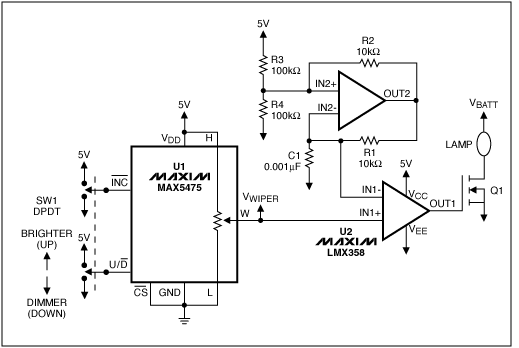# Using a Digital Potentiometer to Implement an Automotive Lighting Dimmer Conserves Power and Increases Efficiency

### Abstract

This application note describes how to use a low-cost digital potentiometer to implement an up/down light-dimming control that both conserves power and increases efficiency.

### Introduction

In automotive and other lighting applications, it is often desirable to dim interior lighting. Using a mechanical potentiometer to perform this function would waste power, be relatively inefficient, and raise concerns about durability. In contrast, the design in this application note leverages user familiarity with up/down cellular phone controls, implementing an up/down light-dimming control that uses a low-cost digital potentiometer (digipot). Using a digipot not only avoids the inefficiency and mechanical durability concerns of a mechanical potentiometer; it actually conserves power and increases efficiency.

### Implementing an Automotive Lighting Dimmer

This design uses a nonvolatile digipot to create a dimming threshold, and it uses a discrete pseudo-saw-tooth oscillator to implement a pulse-width-modulation (PWM) lamp control. The MAX5475 (U1) is a 32-tap nonvolatile digital potentiometer with a 100kΩ end-to-end resistance. The design uses a double-pole/double-throw (DPDT) rocker switch (SW1) to control the digipot. One pole controls the U/D pin of the MAX5475, while the other pole controls the active-low INC pin. Thus, depressing the switch in the "Up" direction causes a high-to-low transition on active-low INC, which increments the digipot; depressing the switch in the "Down" direction decrements the digipot. The nonvolatile nature of the MAX5475's wiper position allows the dimmer setting to be retained in an unpowered state.

The digipot's wiper (VWIPER) is input into the inverting input of an LMX358 (U2) dual operational amplifier (op amp). It is then compared to the pseudo-saw-tooth waveform created by the other half of the dual op amp. Basically, the slope of the saw-tooth ramp creates a PWM drive for FET Q1. Increasing VWIPER increases the duty cycle, the on-time of the FET, and lamp brightness. (In actuality, the ramp is nonlinear as it consists of an RC charging network of R1 and C1. However, over the portion of the charge interval, the waveform is very suitable for a low-cost duty-cycle control.) A logic-level n-channel enhancement FET drives the lamp. Q1 should be selected based on the lamp load requirements.Figure 1. This diagram illustrates how to use the MAX5475 digital potentiometer to implement an automotive lighting dimmer.

To generate the saw-tooth oscillator, the LMX358 is configured as depicted in Figure 1. Resistors R2, R3, and R4 generate the hysteresis of oscillation. With a 5V input, the amp IN2+ input toggles between 0.41V and 4.55V. Note, decreasing the value of R2, increases the overall span of the ramp; increasing the value of R2, reduces the span of the ramp. This design uses an R2 value of 10kΩ to provide a ramp span that utilizes the majority of the resolution of the MAX5475 digital potentiometer. The ramp is created as capacitor C1 charges and discharges between the 0.41V and 4.55V thresholds through resistor R1.

The period of oscillation should be relatively slow to minimize the effect of FET turn-on and turn-off losses. To calculate the period of oscillation (TPERIOD), we must first calculate TDISCHARGE and TCHARGE, where VIN2+(high) = 4.55V and VIN2+(low) = 0.41V.

TDISCHARGE = -R1 × C1 × ln(VIN2+(low)/VIN2+(high)) = 24.1µs(Eq. 1)

TCHARGE = -R1 × C1 × ln{1 - [(VIN2+(high) - VIN2+(low))/(5V - VIN+(low))]} = 32.4µs(Eq. 2)

TPERIOD = TDISCHARGE + TCHARGE = 83.4µs(Eq. 3)

FOSC = 1/TPERIOD = 12kHz

In this case, TPERIOD = 12kHz.

### Summary

This application note illustrates how to use a low-cost digipot to implement a PWM light-dimming control. An up/down control similar to the kind found in cellular phones is used in the design, eliminating the need for a microprocessor, minimizing costs, and leveraging user familiarity with up/down controls. Whereas the use of a mechanical potentiometer to implement an automotive lighting dimmer would raise inefficiency and durability concerns, the use of a digital potentiometer in this design increases efficiency and conserves power.